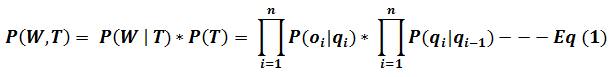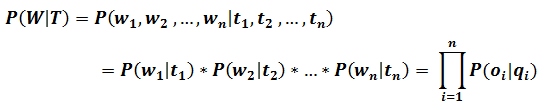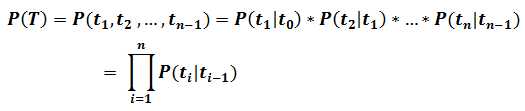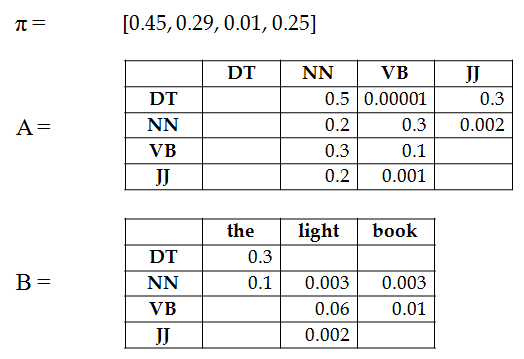Advanced Database Management System - Tutorials and Notes: How to build POS tagging system with bigram Hidden Markov Model?

## Part-Of-Speech Tagging with Hidden Markov Model

### What is POS tagging?

Part-Of-Speech (POS) tagging is the process of attaching each word in an input text with appropriate POS tags like Noun, Verb, Adjective etc. This task is considered as one of the disambiguation tasks in NLP. The reason is, many words in a language may have more than one part-of-speech. You can understand if from the following table;
 Words POS tags Bank Noun, Verb Easy Adjective, Adverb Process Noun, Verb
POS tagging is also called as grammatical tagging or word-category disambiguation.

### Why POS tagging?

It is one of the sub-problems in many NLP applications. For example,

• Machine translation - We need to identify the correct POS tags of input sentence to translate it correctly into another language.

• Word sense disambiguation – Identifying the correct word category would help in improving the sense disambiguation task which is to identify the correct meaning of word.

• Named entity recognition – It is to identify and classify the named entities mentioned in a text. POS tagging is one of the preprocessing steps in here.

Also, there are many other NLP applications that use POS tagging as one of the preliminary steps.

### Different groups of POS tagging techniques

1. Rule based POS taggers
1. Stochastic (Probabilistic) POS taggers

### Hidden Markov Model in POS tagging

HMM is a probabilistic sequence model. POS tagging is one of the sequence labeling problems. A sequence model assigns a label to each component in a sequence. It computes a probability distribution over possible sequences of labels and chooses the best label sequence. POS tagging is a sequence labeling problem because we need to identify and assign each word the correct POS tag.
A hidden Markov model (HMM) allows us to talk about both observed events (words in the input sentence) and hidden events (POS tags) unlike Markov chains (which talks about the probabilities of state sequence which is not hidden).

### Two important assumptions used by HMM

HMM uses two assumptions for simplifying the calculations. They are;
• Markov assumption: the probability of a state qn (POS tag in tagging problem which are hidden) depends only on the previous state qn-1 (POS tag).
P(qn | q1, q2, …, qn-1) = P(qn | qn-1)
• Output independence: the probability of an observation on (words in tagging problem) depends only on the state qn (hidden state) that produced the observation and not on any other states or observations.
P(on | q1,…, qn, …qT, o1, … oi, … oT) = P(on | qn)

### Likelihood of the observation sequence (Forward Algorithm)

Let us consider W as word sequence (observation/emission) and T as tag sequence (hidden states). W consists of a sequence of observations (words) w1, w2, … wn, and T consists of a sequence of hidden states (POS tags) t1, t2, … tn. Then the joint probability, ie., P(W, T) (also called as likelihood estimation) can be calculated using the two assumptions discussed above as follows;Observation (word sequence) probabilities as per the output independence assumptionHOW?State transition (POS tags) probabilities as per Bigram assumption on tags (probability of a tag depends only on its previous tags)HOW?So, Eq 1 can be expanded as follows with observation probabilities followed by transition probabilities;
P(W|T) * P(T) = P(w1|t1) * P(w2|t2) * … * P(wn|tn) * P(t1|t0) * P(t2|t1)
* … * P(tn|tn-1).

### Likelihood estimation - Example:

Question:
Given the HMM λ = (A, B, π) and word sequence W = “the light book”, find P(the light book | Det JJ NN), the probability of the word sequence (observation) given the tag sequence.Solution:
Given are initial state probabilities (π), state transition probabilities (A), and observation probabilities (B).
P(the light book | DT JJ NN)
= P(the|DT) * P(light|JJ) * P(book|NN) * P(DT|start) * P(JJ|DT) * P(NN|JJ)
= 0.3 * 0.002 * 0.003 * 0.45 * 0.3 * 0.2
= 0.0000000486
= 4.86 * 10-8

******************